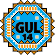General Utility Library for C++14  2.7
Numeric Utilities

The General Utility Library provides several functions dealing with numbers.

#### Modifying Values

abs(): Calculate the absolute value, even for unsigned types.

clamp(): Coerce a value to be within a given range.

#### Greatest Common Divisor & Least Common Multiple

gcd(): Calculate the greatest common divisor of two integers.

lcm(): Calculate the least common multiple of two integers.

#### Converting to Numbers

to_number(): Convert an ASCII string_view into an integer or floating-point number.

#### Approximate Comparisons

within_abs(): Determine if two numbers are almost equal, allowing for an absolute difference.

within_orders(): Determine if two numbers are almost equal, comparing only some significant digits.

within_ulp(): Determine if two numbers are almost equal, allowing for a difference of a given number of units-in-the-last-place (ULPs).

#### Working With Bits in Integral Values

bit_set(): Set a bit in an integral value.

bit_reset(): Clear a bit in an integral value.

bit_flip(): Flip a bit in an integral value.

bit_test(): Test a bit in an integral value.